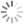Search Filters# 170 results

Showing 1-40 of 170
Sort by     Items per page     Display Grid List RSS Feed
• Gucci pendant 2 3D Model
Gucci pendant 2

• Gucci pendant 3D Model
Gucci pendant

• Ice Age Character Sid 3D Model
Ice Age Character Sid

• Parrot figure 3 3D Model
Parrot figure 3

• Birds figure 3D Model
Birds figure

• Cocker Spaniel figure 3D Model
Cocker Spaniel figure

• Train Horn 3D Model
Train Horn

• Punisher logo 3D Model
Punisher logo

• Shield 18 3D Model
Shield 18

• Agents of shield logo 3D Model
Agents of shield logo

• Chihuahua figure low poly 3D Model
Chihuahua figure low poly

• Elephant figure low poly 3 3D Model
Elephant figure low poly 3

• Shield 20 3D Model
Shield 20

• Christmas decoration 3D Model
Christmas decoration

• Shield 19 3D Model
Shield 19

• Black panther logo 3D Model
Black panther logo

• dog shih tzu figure 2 3D Model
dog shih tzu figure 2

• beagle dog figure 3D Model
beagle dog figure

• cat figure 3 3D Model
cat figure 3

• elephant figure low poly 2 3D Model
elephant figure low poly 2

• dog shih tzu figure 3D Model
dog shih tzu figure

• panda figure 3 3D Model
panda figure 3

• panda figure 2 low poly 3D Model
panda figure 2 low poly

• panda figure low poly 3D Model
panda figure low poly

• dragon figure low poly 3D Model
dragon figure low poly

• girl figure low poly 3D Model
girl figure low poly

• elephant figure low poly 3D Model
elephant figure low poly

• hare figure low poly 3D Model
hare figure low poly

• swan figure low poly 3D Model
swan figure low poly

• ram figure low poly 3D Model
ram figure low poly

• sea horse figure low poly 3D Model
sea horse figure low poly

• Chowchow dog figure 2 3D Model
Chowchow dog figure 2

• bulldog figure 2 3D Model
bulldog figure 2

• Chowchow dog figure low poly 3D Model
Chowchow dog figure low poly

• bulldog figure low poly 3D Model
bulldog figure low poly

• Girl sculpture 3D Model
Girl sculpture 3D print model